cancel
Showing results for
Did you mean:Frequent Visitor

## List Time between the first hour and the last hour

Hi

I have a list of an employee, what hours he worked from to like below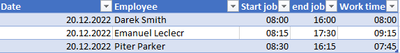I want to get tabel like belowIt is posible in power query? The key columns are Houer and Count.

1 ACCEPTED SOLUTIONSuper User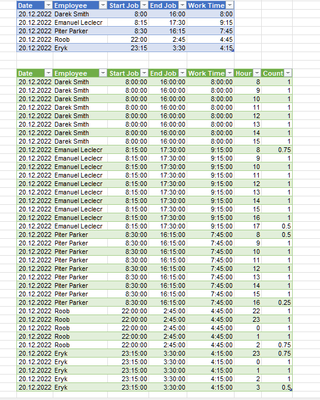``````let
Source = Excel.CurrentWorkbook(){[Name="Table2"]}[Content],
#"Changed Type" = Table.TransformColumnTypes(Source,{{"Date", type date}, {"Employee", type text}, {"Start Job", type time}, {"End Job", type time}, {"Work Time", type time}}),
Custom1 = let fx=(t,d)=>Time.StartOfHour(t)+Duration.From(d/24)
in #table(
Table.ColumnNames(#"Changed Type")&{"Hour","Count"},
List.TransformMany(
Table.ToRows(#"Changed Type"),
(x)=>let Start=DateTime.From(x{2}),
End=DateTime.From(x{3})+Byte.From(x{2}>=x{3})*Duration.From(1)
in
List.Generate(
()=>Start,
each _<End,
each fx(_,1),
each {Time.Hour(_),Duration.TotalHours(List.Min({End,fx(_,1)})-_)}
),
(x,y)=>x&y
)
)
in
Custom1``````
5 REPLIES 5Frequent Visitor

Table like below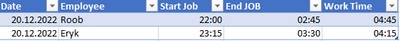ResultsSuper User``````let
Source = Excel.CurrentWorkbook(){[Name="Table2"]}[Content],
#"Changed Type" = Table.TransformColumnTypes(Source,{{"Date", type date}, {"Employee", type text}, {"Start Job", type time}, {"End Job", type time}, {"Work Time", type time}}),
Custom1 = let fx=(t,d)=>Time.StartOfHour(t)+Duration.From(d/24)
in #table(
Table.ColumnNames(#"Changed Type")&{"Hour","Count"},
List.TransformMany(
Table.ToRows(#"Changed Type"),
(x)=>let Start=DateTime.From(x{2}),
End=DateTime.From(x{3})+Byte.From(x{2}>=x{3})*Duration.From(1)
in
List.Generate(
()=>Start,
each _<End,
each fx(_,1),
each {Time.Hour(_),Duration.TotalHours(List.Min({End,fx(_,1)})-_)}
),
(x,y)=>x&y
)
)
in
Custom1``````Frequent Visitor

I found one problem.

For example

When i have
start job on 18:00:00 And end job 02:00:00 ( night shift ) i get errorSuper User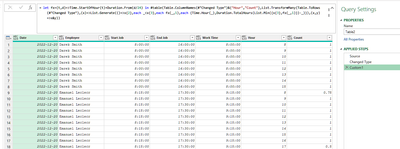``````let
Source = Excel.CurrentWorkbook(){[Name="Table2"]}[Content],
#"Changed Type" = Table.TransformColumnTypes(Source,{{"Date", type date}, {"Employee", type text}, {"Start Job", type time}, {"End Job", type time}, {"Work Time", type time}}),
Custom1 = let fx=(t,d)=>Time.StartOfHour(t)+Duration.From(d/24)
in #table(
Table.ColumnNames(#"Changed Type")&{"Hour","Count"},
List.TransformMany(
Table.ToRows(#"Changed Type"),
(x)=>List.Generate(
()=>x{2},
each _<x{3},
each fx(_,1),
each {Time.Hour(_),Duration.TotalHours(List.Min({x{3},fx(_,1)})-_)}
),
(x,y)=>x&y
)
)
in
Custom1``````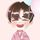Community Support

Yes, we could do such an expection in Power Query.

Here is the whole M syntax:

``````let
Source = Table.FromRows(Json.Document(Binary.Decompress(Binary.FromText("i45WMjLQMzTSMzIwMlLSUXJJLErNVgjOzSzJAPIMLKwMDIC0oRmEhvBjddA0ueYm5pWm5ij4pCbnpCYXQRQamoI0mlsZgzVagvgYGgMyS1KLFAISi7JTobqModaBdRuYW5kAdcUCAA==", BinaryEncoding.Base64), Compression.Deflate)), let _t = ((type nullable text) meta [Serialized.Text = true]) in type table [Date = _t, Employee = _t, #"Start job" = _t, #"end job" = _t, #"Work time" = _t]),
#"Changed Type" = Table.TransformColumnTypes(Source,{{"Date", type text}, {"Employee", type text}, {"Start job", type time}, {"end job", type time}, {"Work time", type time}}),
#"Changed Type with Locale" = Table.TransformColumnTypes(#"Changed Type", {{"Date", type date}}, "en-GB"),
starthour=Time.From(Number.ToText(Time.Hour([Start job]))&":00:00"),
hourdiff=Time.Hour([end job])-Time.Hour([Start job]) +1,
timelist=List.Times(starthour,hourdiff,#duration(0,1,0,0))
][timelist]),
#"Expanded Custom" = Table.ExpandListColumn(#"Added Custom", "Hourer"),
a=Duration.TotalMinutes([Hourer] -[Start job]) ,
b=Duration.TotalMinutes([end job] -[Hourer]),
c=[Start job]>[Hourer],
d= if [end job] <>[Hourer] and b <60 then b/60 else if c then (60+a)/60 else 1
][d]),
#"Extracted Hour" = Table.TransformColumns(#"Added Custom1",{{"Hourer", Time.Hour, Int64.Type}})
in
#"Extracted Hour"``````

Output:Best Regards,

Eyelyn Qin

If this post helps, then please consider Accept it as the solution to help the other members find it more quickly.